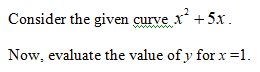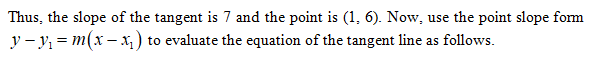# Find the equation of the tangent line to the curve f(x)= x2 +5x when x=1.

Question
1 views

Find the equation of the tangent line to the curve f(x)= x+5x when x=1.

check_circle

Step 1Step 2

Differentiate the given function at substitute x = 1 as follows.

Step 3...

### Want to see the full answer?

See Solution

#### Want to see this answer and more?

Solutions are written by subject experts who are available 24/7. Questions are typically answered within 1 hour.*

See Solution
*Response times may vary by subject and question.
Tagged in

### Other